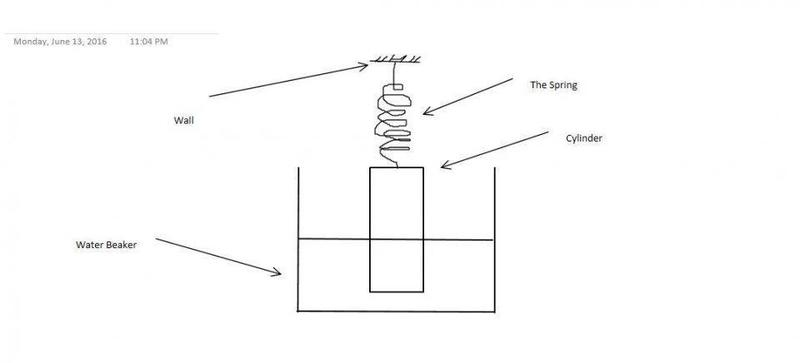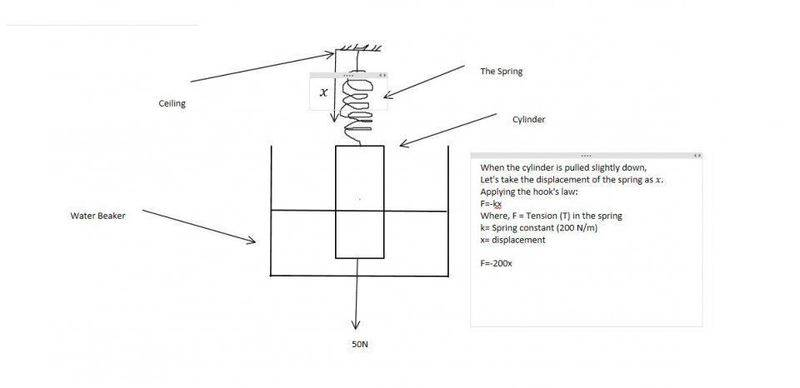# A Simple Harmonic Motion Question

## Homework StatementAs in the given picture, the cylinder is drowned (not completely drowned as in partially drowned) in water. The cylinder is attached with a spring which has the spring constant of 200 N/m. The spring has attached to a unmovable point in the ceiling. The weight of the cylinder is 5 kg and the weight of the spring is inconsiderate. If the cylinder pulled slightly down then will there be a simple harmonic motion and if so what is the Period?

Important note:
The height of the cylinder is not given.
The density of the cylinder is also not given.
The density of the water is 1000 kg/m^3.

## Homework Equations

The hook's law -> F= -kx where F=force
k= the spring constant
x= is the displacement

## The Attempt at a SolutionThe cylinder is moving in accelerated motion. Let's take the acceleration as a.
Applying the Newton's Law:
F=ma regarding upward motion
Where,

F=T-50+U

ma= 5a

Where T= Tension
U= Up thrust

Hence:

T-50+U=5a

-200x-50+U=5a

U=Vdg

Where V= Volume of the cylinder under water
d= Density of the cylinder
g= gravitation acceleration

This is where I am stuck. I cannot identify the volume of the cylinder under water since it is changing during the motion. What should I do next...?

haruspex
Homework Helper
Gold Member
Are you not told the radius of the cylinder?

Are you not told the radius of the cylinder?
Didn't I mention it?

haruspex
Homework Helper
Gold Member
Didn't I mention it?
Ok. When the cylinder is displaced vertically by x (upward positive?) what is the change in the buoyancy force?

Ok. When the cylinder is displaced vertically by x (upward positive?) what is the change in the buoyancy force?
That is the point where I cant understand any further. Please explain...

haruspex
Homework Helper
Gold Member
That is the point where I cant understand any further. Please explain...
What is the change in the volume of water displaced? (If the radius of the beaker is not given then you will have to assume it is much wider than the cylinder.)

How can I calculate it? when there is no height of the cylinder is given...?

haruspex
Homework Helper
Gold Member
How can I calculate it? when there is no height of the cylinder is given...?
We only want the change in the submerged volume for a small change in x. The height of the cylinder will not matter.

So that explains it...
But how can I identify the change in submerged volume?

haruspex
Homework Helper
Gold Member
So that explains it...
But how can I identify the change in submerged volume?
If you push a vertical cylinder radius r into water to a depth of x, what volume is submerged?

That would be
Volume = π*r2*x
Then what?

haruspex
Homework Helper
Gold Member
That would be
Volume = π*r2*x
Then what?
Right. If the submerged volume increases by that much, how much does the buoyancy increase by?

U = Vdg
U = π*r2*x*1000*10
U = 10000πr2x

Then what?

haruspex
Homework Helper
Gold Member
U = Vdg
U = π*r2*x*1000*10
U = 10000πr2x

Then what?
It's better not to plug in numbers until the end. For now, keep it as ##A\rho_wgx##, where A is the cross-sectional area of the cylinder, i.e. ##\pi r^2##.
Next is to write out the force and acceleration equation for the vertical movement of the cylinder. If the cylinder is height x above its equilibrium position, what is the net force acting on it?

•Nipuna Weerasekara
Khashishi
What's the radius/diameter of the beaker?

haruspex
Homework Helper
Gold Member
What's the radius/diameter of the beaker?
See post #3

Khashishi
The radius of the beaker affects how much the water level moves up and down when the cylinder moves.

Edit: I guess it doesn't matter in the end.

Last edited:
•Nipuna Weerasekara
haruspex
Homework Helper
Gold Member
The radius of the beaker affects how much the water level moves up and down when the cylinder moves.

Edit: I guess it doesn't matter in the end.
Sorry, I should have said "see post #6". Yes, I agree that the radius of the beaker matters too, but if it's not given we will have to take it as being effectively infinite.

The problem was solved... The radius of the beaker does not matter nor the height of the cylinder and the density of it.
The method to find the answer is first we have to draw a step of equilibrium of the cylinder.
Then we have to note that the submerged volume of cylinder is h at the equilibrium stage. Whereas the displacement of the spring is noted as c.
Then we can see
F = -kx
F = T
x = c
T = -kc
U = Ahdg
Considering equilibrium of the cylinder:
F= ma
T + U -mg = 0
-kc + Ahdg -mg =0

Then we go to the step where we pull the cylinder for x distance
Then,
Tx = -k (c+x)
F= ma for up
Tx + Ux - mg = ma
Ux = A(h+x)dg
-k (c+x) + A(h+x)dg - mg = ma

-kc -kx + Ahdg +Axdg -mg =ma

-kx + Axdg = ma

The final equation is

a = -(Adg - k)/m * x

Hence the ω2 = (Adg - k)/m
Then ω2 = 2π/t
A = π*0.062 m2 = 0.0036π m2
d = 1000 kgm-3
g = 10 ms-2
k = 200 Nm-1
m = 5 kg
Then t (period of the SHM) can be found...

Thanks...

Last edited:
haruspex
Homework Helper
Gold Member
The radius of the beaker does not matter
It does. Suppose the cylinder has radius r and the beaker has radius R. If the cylinder is displaced upward by x then the spring tension reduces by kx, but the volume of cylinder submerged reduces by ##\pi x\frac{R^2r^2}{R^2-r^2}##.

•Nipuna Weerasekara
Yes that is true but if we use it in this calculation we cannot come to an exact solution like I have came. By the way my teacher said that I should not consider the change in water levels because in this level I don't have to use it in the calculations.
By the way thanks for the valuable advises...

haruspex
•